# Texas Go Math Grade 3 Lesson 13.3 Answer Key Divide by 8

Refer to our Texas Go Math Grade 3 Answer Key Pdf to score good marks in the exams. Test yourself by practicing the problems from Texas Go Math Grade 3 Lesson 13.3 Answer Key Divide by 8.

## Texas Go Math Grade 3 Lesson 13.3 Answer Key Divide by 8

Essential Question
What strategies can you use to divide by 8?

Unlock the Problem
At Stephen’s camping store, firewood is sold in bundles of 8 logs. He has 32 logs to put in bundles. How many bundles of firewood can he make?

• What will Stephen do with the 32 logs?

One Way

Make a model.

You can write a division equation to show how many rows of 8 are in 32.
Look at the array.Count the number of tiles in each row.
How many tiles are there in each row? ___
Show the above array on a grid by shading the squares to record the tiles.
How many rows of squares will you shade? ___
How many in each row will you shade? ___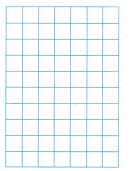So, Stephen can make ___ bundles of firewood.
Write the equation:
___ ÷ ___ = ____

Math Talk
Mathematical Processes
How does knowing 4 × 8 = 32 help you find 32 ÷ 8?

Another Way Use a related multiplication fact.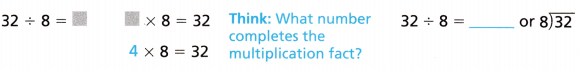Share and Show

Question 1.
Shade the grid to find 16 ÷ 8.
Think: How many rows are shaded?Write the equation:
___ ÷ ___ = ___

Find the unknown factor and quotient.

Question 2.
8 × __ = 56 56 ÷ 8 = ___

Question 3.
___ × 8 = 40 40 ÷ 8 = ___

Find the quotient.

Question 4.
18 ÷ 3 = ___

Question 5.
___ = 48 ÷ 8

Question 6.
56 ÷ 7 = ___

Question 7.
__ = 32 ÷ 8

Problem Solving

Question 8.
Use tools Stephen has a log that is 8 feet long. If he cuts the log into pieces that are 2 feet long, how many pieces will Stephen have?Shade the grid to solve 8 ÷ 2.
Stephen will have ___ pieces.
Write the equation:
__ ÷ __ = ____

Question 9.
H.O.T. Write a word problem that can be solved by using the division equation 18 ÷ 6 = 3.

Algebra Write +, —, ×, or ÷.

Question 10.
6 × 6 = 32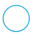4

Question 11.
123 = 19 – 15

Question 12.
40 ÷ 8 = 357

Problem Solving

Use the table for 13-14.

Question 13.
H.O.T. Multi-Step There are 36 people camping at Max’s family reunion. They have cabin tents and vista tents. How many of each type of tent do they need to sleep exactly 36 people if each tent is filled? Explain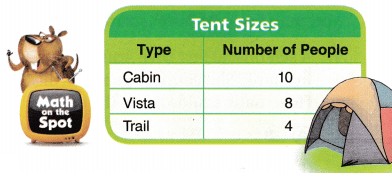Question 14.
There are 32 people who plan to camp over the weekend. What is the least number of trail tents they need? Explain.

Question 15.
Josh is dividing 64 marshmallows equally among 8 campers. How many marshmallows will each camper get?

Question 16.
H.O.T. Representations Grace set 8 plates at each picnic table so 24 campers could eat dinner. How many picnic tables did Grace have to set? Circle the equal groups and write the equation.
___ ÷ __ = ____Write the related multiplication fact.
___ ÷ __ = ____

Fill in the bubble for the correct answer choice.

Question 17.
A scientist at Sea Dive Aquarium is studying squids in a tank. She counts 56 arms in all. Squids have 8 arms. How many squids are in the tank?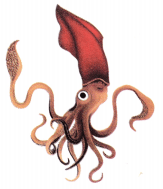(A) 8
(B) 6
(C) 7
(D) 9

Question 18.
The Swansons purchase 48 hotdogs for the family picnic. The hot dogs come in packages of 8. Which equation shows how many packages of hot dogs the Swansons buy?
(A) 48 ÷ 8 = 6
(B) 5 × 8 = 40
(C) 40 ÷ 4 = 10
(D) 7 × 6 = 42

Question 19.
Multi-Step Kyle has 4 packs of baseball cards. Each pack has 12 cards. If Kyle wants to equally divide the cards among him and his 7 friends, how many baseball cards will each person get?
(A) 2
(B) 9
(C) 6
(D) 20

Texas Test Prep

Question 20.
Derek had 64 stickers for his album. He placed 8 stickers on each page. How many pages did he use?
(A) 8
(B) 2
(C) 7
(D) 5

### Texas Go Math Grade 3 Lesson 13.3 Homework and Practice Answer Key

Find the unknown factor and quotient.

Question 1.
8 × __ = 48

Question 2.
__ × 4 = 32

Question 3.
64 ÷ 8 = ___

Question 4.
56 ÷ 8 = __

Find the unknown number.

Question 5.
16 ÷ 8 = ___

Question 6.
40 ÷ __ = 5

Question 7.
9 = __ ÷ 8

Question 8.
__ = 80 ÷ 8

Problem Solving

Question 9.
Ted has a strip of cloth that is 16 feet long. If he cuts the cloth in pieces that are 2 feet long to make kite tails, how many pieces will he have?Shade the grid to solve 16 ÷ 2.
Ted will have ___ pieces.
Write the equation:

Question 10.
There are 40 people going to a baseball game. There are only three vans available that seat 8 people each. The other people will ride in cars that seat 4. How many cars are needed so all 40 people have a ride to the baseball game? Explain.

Question 11.
At the baseball game, the 40 people sit in rows of 8. How many rows are the people seated in?

Texas Test Prep

Lesson Check

Question 12.
Bethany makes 48 party favors. If she puts the favors into 8 baskets, how many favors are in each basket?
(A) 56
(B) 40
(C) 6
(D) 5

Question 13.
Wes has 56 balloons. He uses 8 balloons to make an animal shape. How many animals can Wes make?
(A) 8
(B) 7
(C) 9
(D) 5

Question 14.
Louis has 24 pencils. He has an equal number of red, blue, and yellow pencils. How many pencils of each color does Louis have?
(A) 3
(B) 8
(C) 4
(D) 6

Question 15.
A teacher puts 64 boxes of school supplies on 8 shelves. If she puts an equal number of boxes on each shelf, how many shelves does she need?
(A) 7
(B) 6
(C) 9
(D) 8

Question 16.
Multi-Step Jackie has 15 green tiles and 25 yellow tiles. She divided them equally to decorate 5 plates. How many tiles does she put on each plate?
(A) 3
(B) 7
(C) 8
(D) 5